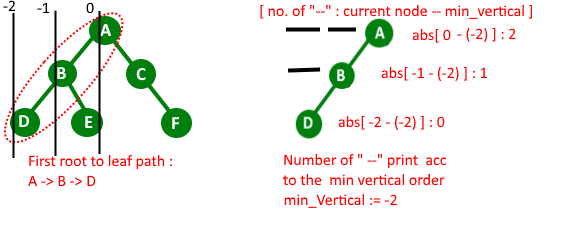# Print all root to leaf paths with there relative positions

Given a binary tree, print the root to the leaf path, but add “_” to indicate the relative position.

Example:

```Input : Root of below tree
A
/   \
B      C
/ \    / \
D   E   F  G

Output : All root to leaf paths
_ _ A
_ B
D

_ A
B
_ E

A
_ C
F

A
_ C
_ _ G
```

## Recommended: Please try your approach on {IDE} first, before moving on to the solution.

Below is complete algorithm :

1) We do Preorder traversal of the given Binary Tree. While traversing the tree, we can recursively calculate horizontal distances or HDs. We initially pass the horizontal distance as 0 for root. For left subtree, we pass the Horizontal Distance as Horizontal distance of root minus 1. For right subtree, we pass the Horizontal Distance as Horizontal Distance of root plus 1. For every HD value, we maintain a list of nodes in a vector (” that will store information of current node horizontal distance and key value of root “).we also maintain the order of node (order in which they appear in path from root to leaf). for maintaining the order,here we used vector.
2) While we reach to leaf node during traverse we print that path with underscore “_”
Print_Path_with_underscore function……a) First find the minimum Horizontal distance of the current path.
……b) After that we traverse current path
……….First Print number of underscore “_” : abs (current_node_HD – minimum-HD)
……….Print current node value.

We do this process for all root to leaf path

Below is C++ implementations of above idea.

 `// C++ program to print all root to leaf paths ` `// with there relative position ` `#include ` `using` `namespace` `std; ` ` `  `#define MAX_PATH_SIZE 1000 ` ` `  `// tree structure ` `struct` `Node ` `{ ` `    ``char` `data; ` `    ``Node *left, *right; ` `}; ` ` `  `// function create new node ` `Node * newNode(``char` `data) ` `{ ` `    ``struct` `Node *temp = ``new` `Node; ` `    ``temp->data = data; ` `    ``temp->left = temp->right = NULL; ` `    ``return` `temp; ` `} ` ` `  `// store path information ` `struct` `PATH ` `{ ` `    ``int`  `Hd; ``// horigontal distance of node from root. ` `    ``char` `key; ``// store key ` `}; ` ` `  `// Prints given root to leaf path with underscores ` `void` `printPath(vector < PATH > path, ``int` `size) ` `{ ` `    ``// Find the minimum horizontal distance value ` `    ``// in current root to leaf path ` `    ``int` `minimum_Hd = INT_MAX; ` ` `  `    ``PATH p; ` ` `  `    ``// find minimum horizontal distance ` `    ``for` `(``int` `it=0; it &AllPath, ` `                       ``int` `HD, ``int` `order ) ` `{ ` `    ``// base case ` `    ``if``(root == NULL) ` `        ``return``; ` ` `  `    ``// leaf node ` `    ``if` `(root->left == NULL && root->right == NULL) ` `    ``{ ` `        ``// add leaf node and then print path ` `        ``AllPath[order] = (PATH { HD, root->data }); ` `        ``printPath(AllPath, order+1); ` `        ``return``; ` `    ``} ` ` `  `    ``// store current path information ` `    ``AllPath[order] = (PATH { HD, root->data }); ` ` `  `    ``// call left sub_tree ` `    ``printAllPathsUtil(root->left, AllPath, HD-1, order+1); ` ` `  `    ``//call left sub_tree ` `    ``printAllPathsUtil(root->right, AllPath, HD+1, order+1); ` `} ` ` `  `void` `printAllPaths(Node *root) ` `{ ` `    ``// base case ` `    ``if` `(root == NULL) ` `        ``return``; ` ` `  `    ``vector Allpaths(MAX_PATH_SIZE); ` `    ``printAllPathsUtil(root, Allpaths, 0, 0); ` `} ` ` `  `// Driver program to test above function ` `int` `main() ` `{ ` `    ``Node *root = newNode(``'A'``); ` `    ``root->left = newNode(``'B'``); ` `    ``root->right = newNode(``'C'``); ` `    ``root->left->left = newNode(``'D'``); ` `    ``root->left->right = newNode(``'E'``); ` `    ``root->right->left = newNode(``'F'``); ` `    ``root->right->right = newNode(``'G'``); ` `    ``printAllPaths(root); ` `    ``return` `0; ` `} `

Output:

```_ _ A
_ B
D
==============================
_ A
B
_ E
==============================
A
_ C
F
==============================
A
_ C
_ _ G
```

This article is contributed by Nishant Singh. If you like GeeksforGeeks and would like to contribute, you can also write an article using contribute.geeksforgeeks.org or mail your article to contribute@geeksforgeeks.org. See your article appearing on the GeeksforGeeks main page and help other Geeks.

My Personal Notes arrow_drop_up

Improved By : ManasChhabra2

Article Tags :
Practice Tags :

1

Please write to us at contribute@geeksforgeeks.org to report any issue with the above content.